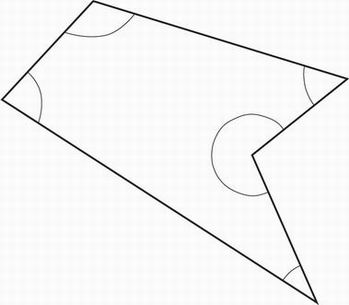# SAT Math Multiple Choice Question 668: Answer and Explanation

### Test Information

Question: 668

8.The figure above shows a polygon with five sides. What is the average (arithmetic mean) of the measures, in degrees, of the five angles shown?

• A. 108°
• B. 110°
• C. 112°
• D. 114°